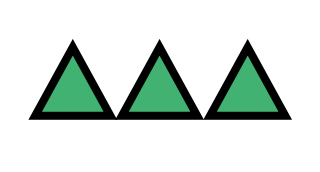Multiplication Review
Multiplication Properties
Factors
Prime and Composite Numbers
Multiples
Inverse Operations
100

What would the multiplication problem look like for

7 + 7 + 7 + 7

7 x 4

100

Is 7 x 0 = 0 an example of the Zero Property?

Yes, 7 x 0 = 0 is an example of the Zero Property.

100
How can we check our answers after finding a number factors?

Factor Rainbow

100

What is a Prime Number?

A Prime Number is a number that only has two factors, 1 and itself.

100

How are we counting when we are naming multiples of a number?

Skip Counting

100

What is the Inverse Operation of addition?

Subtraction
200

What are the numbers that you multiply together called?

Factors

200

What is the Identity Property?

Any number multiplied by ONE is always the original number

200

When trying to find the factors of a bigger number what operation can we use to find them?

Division

200

What is a Composite Number?

Number with multiple factors.

200

Is 25 a multiple of 5?

Yes because 5 x 5 is 25.
200
What is the Inverse Operation of Multiplication?

Division

300

What is the answer to a multiplication problem called?

Product

300

What is the Commutative Property?

The Commutative Property of multiplication means the order of the numbers does not change the result (answer) of the problem.

300

Is 5 a factor if 75?

Yes because 5 x 15 is 75.

300

Is 45 a prime or a composite number?

Composite

300

Is 30 a multiple of 6?

Yes because 6 x 5 is 30.

300

What is the Inverse Operation of Division?

Multiplication

400

What multiplication problem does this array show?3 x 1

400

What is the Associative Property?

The Associative Property tells us that it does not matter the groupings of factors in an equation, the answer will be the same

400

Find the factors for the number 18

1, 2, 3, 6, 9, 18

400

Name a composite number.

4, 6, 8, 9, 10, 12, 14, 15, 16, 18, 20, 21, 22, 24, 25, 26, 28, 30, 32, 33, 34, 35, 36, 38, 39, 40...
400

Is 72 a multiple of 9?

Yes because 9 x 8 is 72.

400

What is the Inverse Operation for the equation 5 x 4 = 20

20 ÷ 4 = 5

20 ÷ 5 = 4

500

Draw an Array for the multiplication problem 4 x 6.500

What is the Distributive Property?

In the Distributive Property, you distribute, pass, or hand out multiplication to numbers within parenthesis using addition or subtraction.

500

Find the factors for the number 30

1, 2, 3, 5, 6, 10, 15, 30

500

Name a Prime Number.

2, 3, 5, 7, 11, 13, 17, 19, 23, 27, 29, 31, 37, 39, 41, 43, 47...

500

How is multiple different than a factor?

a factor is the numbers multiplied together to find a product

a multiple is the numbers we get when skip counting

500

What is the Inverse Operation of the equation 63 ÷ 9 = 7?

9 x 7 = 63

7 x 9 = 63

Click to zoom